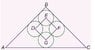# Geometry with circles, triangles and squares

• thereddevils

## Homework Statement

The diagram below shows four congruent circles whose centres are the vertices of the square DEFG and whose circumference touch the sides of an isosceles triangle. Area of triangle ABC is 10000 units square. What is the radius of the circles, to the nearest unit.

## The Attempt at a Solution

Let the sides AB=BC=x and the radius, r.

1/2 x^2=10000 so x is approximately 142

AC = 141 sqrt(2)

I assumed that the height of the triangle ABC passes through BEG and the point where the bottom circle touches AC. Maybe this is why i arrived at the wrong answer.

(1/2)(r) (141 sqrt(2))(1+sqrt(8)+sqrt(2))=10000

r=10

But the answer given is 19.

## Homework Statement

The diagram below shows four congruent circles whose centres are the vertices of the square DEFG and whose circumference touch the sides of an isosceles triangle. Area of triangle ABC is 10000 units square. What is the radius of the circles, to the nearest unit.

## The Attempt at a Solution

Let the sides AB=BC=x and the radius, r.

1/2 x^2=10000 so x is approximately 142

AC = 141 sqrt(2)

I assumed that the height of the triangle ABC passes through BEG and the point where the bottom circle touches AC. Maybe this is why i arrived at the wrong answer.

(1/2)(r) (141 sqrt(2))(1+sqrt(8)+sqrt(2))=10000

r=10

But the answer given is 19.

So where's the diagram? I can't think of where exactly the isosceles triangle might be, so a picture or description would help.

Oops, sorry here it is.

#### Attachments

•circles inscribed in triangle.jpg
12.8 KB · Views: 434

In your first step, A = 1/2 x^2 only works if angle B is 90 degrees.

Can you find the measures of [itex]\angle A[/tex], [itex]\angle B[/tex], and [itex]\angle C[/tex]?

Can you find the measures of [itex]\angle A[/tex], [itex]\angle B[/tex], and [itex]\angle C[/tex]?

Isn't that the small triangle(draw a diagonal in the square) similar to triangle ABC?In that case angle B is 90 degree.

Well, this is my thought process.

Consider the first circle(uppermost one), draw lines connecting point E and the two tangent points call them T1 and T2.

Shouldn't T1 E = B T2 and E T2 = B T1? In this case, triangle BT1 E and B T2 E are isosceles triangles and angle B is 90 degree.Anyways, it needs to be verified. Thanks.

Yes, [itex]\angle B ~=~ 90^\circ[/tex]

It should be obvious by inspection, but you know that given 2 circles that intersect at only one point, a line that is tangent to both must be parallel to the line through their centers. Then, given that DEFG is square, you have ED [itex]\perp[/tex] EF, so AB [itex]\perp[/tex] BC.

So, what do [itex]\angle A[/tex] and [itex]\angle C[/tex] measure?

Yes, [itex]\angle B ~=~ 90^\circ[/tex]

It should be obvious by inspection, but you know that given 2 circles that intersect at only one point, a line that is tangent to both must be parallel to the line through their centers. Then, given that DEFG is square, you have ED [itex]\perp[/tex] EF, so AB [itex]\perp[/tex] BC.

So, what do [itex]\angle A[/tex] and [itex]\angle C[/tex] measure?

ohoh! what a mistake! i was already correct in my first post. It's an arithmetic error in the final step. The answer i got is 19.13 which is approximately 19.

Last edited:

ohoh! what a mistake! i was already correct in my first post. It's an arithmetic error in the final step. The answer i got is 19.13 which is approximately 19.

I didn't check your work since I wasn't sure how you arrived at those values.
I come up with approx. 19.07 (still 19 when rounded)

I didn't check your work since I wasn't sure how you arrived at those values.
I come up with approx. 19.07 (still 19 when rounded)

Thanks zgozvrm, would you mind posting your solution?

It's basically the same as what you've got. My point was that it wasn't evident how you got those numbers. (I get approx. 19.07 with your equation too, if I don't round off the intermediate steps).

So here's my solution, with justifications:

Like you said, we have [itex]\angle B = 90^\circ[/tex] and, with [itex]\triangle ABC[/tex] being isosceles, we have [itex]\angle A = \angle C = 45^\circ[/tex] and $$\overline{AB} \, = \, \overline{BC}$$

Drawing the height of [itex]\triangle ABC[/tex] from B perpendicular to $$\overline{AC}$$ intersecting at point H (the tangent point with circle G), we will have a line segment that bisects circles E and G through their centers. Therefore, the height of [itex]\triangle ABC[/tex] is equal to the length of the diagonal of square DEFG (which is $$\overline{EG}$$) plus the radius of circle G (which is $$\overline{GH}$$) plus the length of $$\overline{BE}$$ (which can be shown to be equal to half the length of $$\overline{EG}$$).

Being an isosceles triangle, this height $$\overline{BH}$$ will bisect [itex]\angle B[/tex], forming a new isosceles triangle [itex]\triangle ABH[/tex] having $$\overline{AH} \, = \, \overline{BH}$$

But (like you stated), we have the area of

$$\triangle ABC = \frac{1}{2} \overline{AB} \times \overline{BC} = \frac{1}{2} \overline{AB}^2 = 10,000$$

This gives us

$$\overline{AB} = \sqrt{20,000} = 100 \sqrt2 \approx 141.4$$

But this is the hypotenuse of [itex]\triangle ABH[/tex], so we have $$\overline{AH} \displaystyle ^2 +~ \overline{BH} \displaystyle ^2 = 2 \times\overline{BH} \displaystyle ^2 = 20,000$$ and $$\overline{BH} \displaystyle= 100$$

Call R the radius of the circles. Then you get the length of a side of square DEFG equal to 2R and it's diagnonal (say, $$\overline{EG}$$ equal to [itex]\sqrt{(8R^2)} = 2R \sqrt(2)[/tex]

So,

$$\overline{EG} = 2R \sqrt2$$

$$\overline{BE} = \textstyle \frac{1}{2} \displaystyle \overline{EG} = R \sqrt2$$

$$\overline{GH} = R$$

Therefore,

$$\overline{BH} = 2R \sqrt2 ~+~ R \sqrt2 ~+~ R = 3R \sqrt2 ~+~ R = \overline{AH} = 100$$

Solving for R,

$$R \times \left( 3 \sqrt2 \, + 1 \right) = 100$$

$$R = \frac{100}{3 \sqrt2 \, + 1} \approx 19.07$$